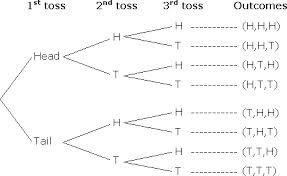# GCSE Maths – Tree Diagrams

A tree diagram is a diagram that is used to help us see all possible outcomes of an event and then calculate their probabilities. Each branch in a tree diagram represents a possible outcome and to find the probability of that particular outcome we multiply together all the individual probabilities along that branch.

Example

A coin is tossed three times, falling either ‘Heads’ or ‘Tails’. Construct the tree diagram.NB: If the probabilities at each toss are P(head) = h, P(tail) = t [NB: t = 1-h]

Then... P(H,H,H) = h x h x h =h3

P(H,H,T) = P(H,T,H) = P(T,H,H) = h x h x t  =h2

P(T,T,H) = P(T,H,T) = P(H,T,T) = t x t  x h = t 2h

P(T,T,T) =  t x t x t = t

Sample question

What are the probabilities of getting a) exactly 2 Heads and b) at least 1 Head

If i) the coin is fair [i.e. P(Head) = P(Tail) = 0.5]?  ii) the coin is unfair, with P(Head) = 0.4?

In part i) h = t  = 0.5, and in part ii) h = 0.4, so t  = 1 – 0.4 = 0.6, and in both parts:

a) P(exactly 2 Heads) = P(H,H,T) + P(H,T,H) + P(T,H,H) = 3 x P(H,H,T) =3h2

b) P(at least 1 Head) = 1 – P(no Heads) = 1 – P(3 Tails) = 1 - t 3

i) a) P(2 Heads) = 3(0.5)2(0.5) = 0.375, b) P(at least 1 Head) = 1 - (0.5)3 = 0.875

ii) a) P(2 Heads) = 3(0.4)2(0.6) = 0.288, b) P(at least 1 Head) = 1 - (0.6)3 = 0.784

### Work Towards That 'A' Grade

Achieve the results you want with-written revision resources when you register with iRevise.
Its Free!